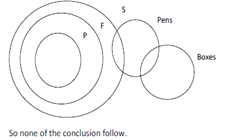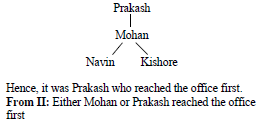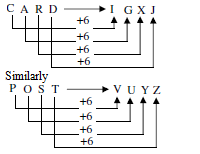# Lion Bridge Reasoning Question

1. In a certain code SPIDERMAN is coded as '954712863' . How is SEMINAR written in that code?
1. 9184362
2. 7617942
3. 7854352
4. 4539861
5. None of these
Every alphabet in SEMINAR is also present in SPIDERMAN. Therefore, if in a certain code an alphabet is coded by a number, it remains the same for other word also.
Here, S is coded as 9, P is coded as 5 and so on.
Therefore, code for SEMINAR is 9184362.
Given an input line; the machine arranges the words and numbers in steps in a systematic manner as illustrated afterwards : Study the pattern and answer the question that follows.
Given an input line, a coding machine rearranges the input following certain steps as explained below:
Input: 47 desert go 56 there often 32 12
Step I: there 47 desert go 56 often 32 12
Step II: there 12 47 desert go 56 often 32
Step III: there 12 often 47 desert go 56 32
Step IV: there 12 often 32 47 desert go 56
Step V: there 12 often 32 go 47 desert 56
2. Which step will be the last step for the input '27 sports 48 television commentary 18 house 36'?
1. IV
2. V
3. VI
4. VII
5. None of these
The logic followed in the given sequence is " numbers are arranged in increasing order while words are arranged in decreasing order of the first alphabet "
Input: 27 sports 48 television commentary 18 house 36
Step I: 27 sports 48 television commentary 18 house 36
Step II: television 27 sports 48 commentary 18 house 36
Step III: television 18 sports 27 48 commentary house 36
Step IV: television 18 sports 27 house 48 commentary 36
Step V: television 18 sports 27 house 36 48 commentary
Step VI: television 18 sports 27 house 36 commentary 48
Here, the complete arrangement is obtained in step VI. Hence, Step VI is the last step.
DIRECTIONS for the question 3: The question below contains four statements, followed by four conclusions numbered I, II, III & IV. You have to take the given statements to be true even if they seem to be at variance with commonly known facts. Read all the conclusions and then decide which of the given conclusions logically follows from the given statements disregarding commonly known facts.
1. Statements:
a.All poles are fans.
b. All fans are stands.
c.Some stands are pens.
d.Some pens are boxes.
Conclusions:
I. Some boxes are poles.
II. Some fans are boxes.
III. Some pens are poles.
IV. Some pens are fans.
1. None follows
2. Only I and II follow
3. Only II and IV follow
4. Only III and IV follow
5. Only I, II and IV followI. Some boxes are poles : This is cleary false.
II. Some fans are boxes :This is cleary not true.
III. Some pens are poles: This is cleary false.
IV. Some pens are fans : This statement is not true.
Therefore, correct answer is option A.
DIRECTIONS for the question 4 to 5: The question below is followed by two statements marked I and II. Mark as your answer.
1. if the data in statement I alone are suffi­cient to answer the question, while the data in statement II alone are not sufficient to answer the question.
2. if the data in statement II alone are suf­ficient to answer the question, while the data in state­ment I atone are not sufficient to answer the question.
3. if the data either in statement I alone or in statement II alone are sufficient to answer the ques-tion.
4. if the data given in both the statements I and II together are not sufficient to answer the ques­tion; and
5. if the data in both the statements 1 and II together are necessary to answer the question.
2. How many sons does K have?
I. M and T are brothers of D.
II. D is the only daughter of N and K.

I alone is not sufficient because it lacks information regarding K. II alone is not suffi­cient because it does not give information about the number of sons (of K).
From I and II: We get that M and T are sons of K. Thus, K has either two or more than two sons.
Since our answer is not clear, both I and II even together are not sufficient.
3. Who among Navin, Mohan, Prakash and Kishore reached office first?
I. Mohan reached office before Navin and Kishore but was not the first to reach office.
II. Kishore reached office after Mohan but before Navin.

From I:Hence data in statement I alone are suffi¬cient to answer the question, while the data in statement II alone are not sufficient to answer the question. so option A is the answer.
DIRECTIONS for the question 6: Solve the following question and mark the best possible option.
'P # Q' means 'P is neither greater than nor equal to Q'.
'P © Q' means' P is neither equal to nor smaller than Q'.
'P % Q' means 'P is neither smaller than nor greater than Q’.
'P $Q' means' P is not smaller than Q'. 'P @ Q' means 'P is not greater than Q'. Now in each of the following questions, assuming the given statements to be true, find which of the three conclu¬sions I, II and III given below them is/are definitely true and give your answer accordingly. 4. Statements: H$V, V%M, K©M
Conclusions:
II. M@H
1. Only I and III are true
2. Only II and III are true
3. Only I and II are true
4. All are true
5. None of these
Here we have
H > V…(i)
V = M….(ii)
K > M….(iii)
From (i) and (ii), we get H > V = M ….(iv)
From (ii) and (iii), we get K > M = V…..(v)
Now, from (v) we get K > V (conclusion I). Hence, conclusion I follows. Again, from (iv), we get M < H (conclusion II). Hence, conclu¬sion II follows. But from (iv) and (v), we can't get any specific relationship between H and K. Hence, conclusion III (H > K) does not follow.
Therefore, Only I and II are true.
DIRECTIONS for the question 7 to 9: Solve the following question and mark the best possible option.
5. Which of the following symbols should be placed in the blank spaces respectively (in the same order from left to right) in order to complete the given expression in such a manner that makes the expressions 'B > N’ as well as 'D < L’ definitely true?
B L O N D
1. =, =, >=, >=
2. >, >=, =, >
3. >, < , =, >=
4. > , =, =, >=
5. > , =, >=,>
We want expressions 'B > N’ as well as 'D < L’ definitely true in B L O N D Going options wise: Option 1 : B = L = O N D In the above expression, we have B N and D L So, it's incorrect. Option 2 : B > L O = N > D In the above expression, B > N and D < L. So, it's incorrect. Option 3 : B > L < O = N D< div=""> In the above expression, relationship between B and N cannot be determined. Option 4 : B > L = O = N D In the above expression, we have B > N and D L<>. Hence, the correct answer is option D.
6. Which of the following should be placed in the blank spaces respectively (in the same order from left to right) in order to complete the given expression in such a manner that makes the expression 'A < P’ definitely false ?
________ < _________<________>_________
1. L, N, P, A
2. L, A, P, N
3. A, L, P, N
4. N, A, P, L
5. P, N, A, L
If we go by the options, using option 1:
L N < P > A
This relations assures us that P > A and is true.
Therefore, what we require “ ‘A < P ’ definitely false,” goes wrong here.
Now, use option 5,
P N < A > L
This relation tells us that N might be greater than or equal to P but N is definitely smaller than A, Therefore, A is definitely larger than P.
This makes the relation A < P definitely false and hence is the required answer.
7. Which of the following symbols should be placed in the blank spaces respectively (in the same order from left to right) in order to complete the given expression in such a manner that makes the expression 'F > N' and 'U > D' definitely false?
F ____O____U_____N_____D
1. <. <, >, =
2. <, =, =, >
3. <, =, =. <
4. >=, =, =,>=
5. >, >, =, <
Expression 'F > N' and 'U > D' definitely false is the condition.
By going options wise :
Option 1 : FN=D
In the above expression, the relationship between F and N cannot be found.
Option 2 : FD
In the above expression, FD
Option 3 : FIn the above expression, FSo, F>N and U>D are definitely false.
So, the correct answer is option C.
8. CARD: IGXJ: : POST: ?
1. UTXY
2. VUYZ
3. UTYZ
4. VUXY